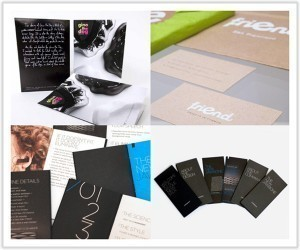•• # Photo Print Sizes

Photo print sizes are marked with an nR, where n denotes the length of theshort edge in inches. Below are some common sizes employed in the United States and other nations.

### Common Print Dimensions

Size 3¼" × 4½ is equal to 82.5 × 120 mm and has a ratio of 16:11 (1.45). In Japan the size is called E. The 9 cm x 13 cm measures 3½" × 5" or 89 × 127 mm (ratio 10:7 (1.43). The print is 3R.

The 4R print (in Japan, KG) measures 4" × 6" or 102 × 152 mm. The ratio is 3:2 (1.5). Often referred to as 10 cm x 15 cm, it is the standard photo print size in the Canada and the United States.

Print 4D measures 4½" × 6" and has an aspect ratio of 4:3. This size is used mainly by digital cameras. The 5R photo print is 5" × 7" (equal to 127 × 178 mm). Called 2L in Japan, its aspect ratio is 7:5 (1.4). This is referred to as 13 cm by 18 cm in many countries. It is double the size of the 3R.

### Other Print Sizes

The 6R photo print is 6" × 8" (equivalent to 152 × 203 mm). The aspect ratio is 4:3 (1.33). In Japan it is called the 8P. It is double the dimensions of the 4R.

The 8R photo print size is 8" × 10" (203 × 254 mm). The aspect ratio is 5:4 (1.25). This is called the 6P in Japan. In other countries it is known as 20 x 25 cm. This size is utilized for 8 x 10 mm film contact prints.

The S8R (6PW in Japan) is 8" × 12" (equivalent to 203 × 305 mm). The aspect ratio is 3:2 (1.5). It is referred to as 20 cm x 30 cm by consumers. It is close to the A4 size and double that of the 6R.

The photo print size 10R measures 10" × 12" (254 × 305 mm). The aspect ratio is 6:5 (1.2); it is known as 4P in Japan. The S10R measures 10" × 15" (or 254 × 381 mm); its aspect ratio is 3:2 (1.5). This is the Japanese 4PW. The 11R size is 11" × 14" (279 × 356 mm). Referred to as the 28 cm by 39 cm, its aspect ratio is 14:11 (1.27).

The S11R measures 11" × 17"; that is 279 × 432 mm. The aspect ratio is 17:11 (1.54). The 12R measures 12" × 15" or 305 × 381 mm, with an aspect ratio of 5:4 (1.25). The S12R is 12" × 18" (305 × 465 mm), and the aspect ratio is 3:2 (1.5).

### A Note on Scaling a Photo Print

Because the aspect ratios of prints vary, scaling can be difficult. Check the measurements you’re using to see if it is possible.
When choosing photo print sizes, centimeters may be used instead. Also, some prints use the Super size, which is popular with 135 mm films.